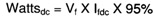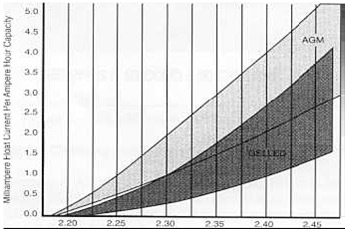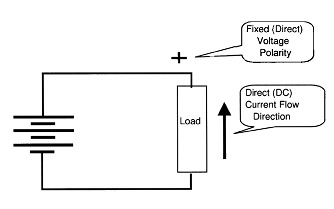Charger Output AC Ripple Voltage and Affect on VRLA Batteries
Click:4686　Date:2008-12-27 00:00:00　Information Source:

The operating temperature is a major factor in the determination of a VRLA battery's service life. The battery temperature is a function of both the temperature of the operating environment and the heat generated within the battery. Heat will be generated within the battery due to exothermic electrochemical reactions within the cells and current, both DC and AC, passing through the resistive components of the cells  .

During float charging of the battery with a pure DC voltage (no AC ripple), the total energy input to the cell is expressed in watts and calculated as the float charging voltage  multiplied by the float charging current (If). For example, if a fully charged VRLA battery was accepting 0.2 amperes while float charged at 13.8 vdc it would be consuming 2.76 watts
from the charger.

The self-discharge rate of the VRLA battery is very small and only about 2% of the float current will be consumed in offsetting the self-discharge reactions. Under normal conditions, another 2% to 3 % is consumed in the overcharge grid corrosion and gassing reactions. The bulk of the float charging current or power (up to 96%) is consumed in the oxygen recombination cycle. The oxygen recombination cycle is an exothermic reaction and it generates heat in addition to that resulting from the passage of the float current through the resistive components of the battery. Therefore, it is safe to assume that up to 95% of the float charging DC power is consumed and dissipated as heat - so the watts dissipated as heat would be:DC Float Voltage, Float Current and Heating
The DC float current is actually quite small and will naturally vary with the charging voltage as is shown in Figure 1. Note that the float current varies with not only the float voltage but also with the type of VRLA battery technology (AGM vs. gel). This reflects in large part the differences in the efficiencies of the oxygen recombination cycle of the two technologies. For example, a 100 Ah AGM VRLA battery draws 200 milli-amperes (0.2 amperes) when charged at 2.30 volts DC per cell while the gel VRLA equivalent battery will only draw 100 milli-amperes. As a result, the heat generated by the AGM battery will also be greater.Figure - VRLA Battery Float Current vs. Voltage
The 100 Ah capacity AGM battery, drawing 0.2 amperes with a charging voltage of 13.8 vdc would develop as a maximum, 2. 6 watts (0.2 amperes X 13.8 vdc X 95%). In contrast the 100
Ah gel VRLA battery drawing 0.1 amperes could only generate 1.3 watts (0.1 Amperes X 13.8 X 95% vdc) of heat.
Heat is generally expressed in terms of British Thermal Units (BTU's) which is the amount of heat required to heat one pound of water from 5g°F to 60°F. One watt of heat will generate
3.41 29 BTU's of heat per hour. Therefore;
(AGM) 2.6 watts X 3.41 29 BTU per hour/watt = 8.87 BTU per hour per 12 volt battery
(Gel) 1.3 watts X 3.4129 BTU per hour/watt = 4.435 BTU per hour per 12 volt battery

Lead Acid Battery and Direct Current Flow
The lead acid battery is an electro-chemical device that produces a constant polarity and amplitude of voltage resulting in a constant direct flow of current (DC or direct current) in a single direction when applied to a resistive load.Figure 2 - Direct Current Flow from a Battery
The lead acid battery produces a DC voltage and current on discharge as noted in Figure 2. Likewise, it requires a DC voltage and current for recharging. However, the DC power required for charging is normally generated by converting the commercial AC power to an acceptable form of DC power.

[ Print ]     [ Close ]